# The Boolean Way — It’s Logical!Electronics guide > Digital integrated circuits I > The Boolean Way — It’s Logical!

Now we already know that the Boolean statement for a NAND gate is: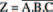But we can also derive another Boolean statement from the above circuit, by the very fact that the inputs to the OR gate in Figure 10.42 are inverted before they reach the OR gate, such that the final output Z is equal to logic 1 when NOT A OR NOT B OR NOT C are logic 1.

So, the Boolean statement: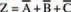also expresses the NAND gate.

In other words: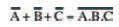NOR from AND and NOT

And, just for the sake of completion, we can also work out that a NOR gate can be created by inverting the inputs of an AND gate.

As we’ve done so far in this book, we’ll do this first experimentally, building up the circuit and checking results. Then we’ll do it mathematically.

Figure 10.43 shows a circuit we can use to do this. It’s basically a two input AND gate, the inputs of which have been inverted by NOT gates.

Figure 10.44 shows a possible breadboard layout you can follow to build the experimental circuit on, while Figure 10.45 is an uncompleted truth table for you to complete with your results, and Figure 10.46 is the complete truth table whose results should match yours.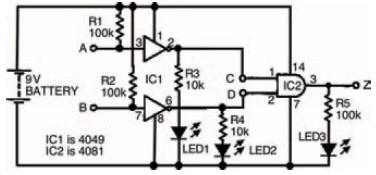Figure 10.43 The experimental circuit to prove that a NOR gate can be created from an AND gate whose inputs are inverted first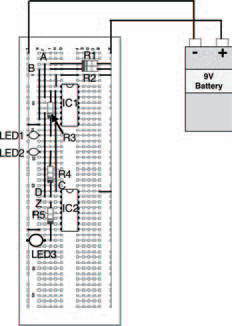Figure 10.44 A breadboard layout for the circuit in Figure 10.43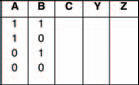Figure 10.45 An incomplete truth table for you to record your results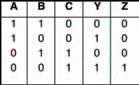Figure 10.46 A completed truth table for the circuit in Figure 10.43 — showing how we have made a NOR gate from an AND gate with NOT gates at its inputs

And, for the sake of completeness, Figure 10.47 shows a three input AND gate with NOT gates at its inputs, and the circuit’s truth table, to show that we can make a NOR gate of any number if inputs from an AND gate of that number of inputs together with the requisite number of inverters.

On the other hand, of course, and even better than this, by the same means of deduction we used for other circuits combining logic gates, we can calculate that the standard NOR Boolean statement: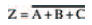is also the same as another Boolean statement: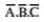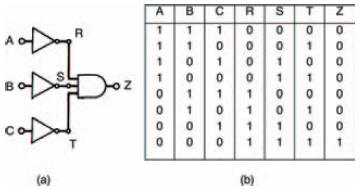Figure 10.47 A three input AND gate converted to a NOR gate with NOT gates

In other words: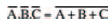And — there — we have just proved mathematically that you can make a NOR gate from AND and NOT gates.

So, there’s no doubt about it, all digital electronic logic gates can be created from a number of other digital electronic logic gates.

What’s more, it’s not beyond the realms of possibility to work out for ourselves that these very logic gates, that can make any other logic gates, can be combined, and combined again, to make up ever more complex circuits. And, indeed they are — as we’ll see in the next chapter! For now, have a go at the quiz over the page, to see if you’ve been taking notice…

<< That ol’ black magic!